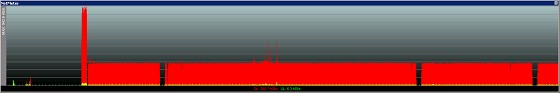Since last Friday I have been getting this weird issue where I will be browsing a web site or streaming a YouTube video and through put will just halt so the page will timeout or the video will stop streaming. The connection stays up and I am am able to ping the site in question and an Xnet server.

For example below is a screenshot of data when streaming a YouTube video. As you can see the data just stops in places. Pings during this time seem to get higher from the YouTube server I'm streaming from but not from a local Xnet hopLarge size here: https://cdn.geekzone.co.nz/imagessubs/e868283f1019aa3ab9bc5cb1c29139df.jpg

Reply from 74.125.237.7: bytes=32 time=89ms TTL=54
Reply from 74.125.237.7: bytes=32 time=89ms TTL=54
Reply from 74.125.237.7: bytes=32 time=90ms TTL=54
Reply from 74.125.237.7: bytes=32 time=89ms TTL=54
Reply from 74.125.237.7: bytes=32 time=91ms TTL=54
Reply from 74.125.237.7: bytes=32 time=279ms TTL=48
Reply from 74.125.237.7: bytes=32 time=280ms TTL=48
Reply from 74.125.237.7: bytes=32 time=280ms TTL=48
Reply from 74.125.237.7: bytes=32 time=283ms TTL=48
Reply from 74.125.237.7: bytes=32 time=281ms TTL=48
Reply from 74.125.237.7: bytes=32 time=283ms TTL=48
Reply from 74.125.237.7: bytes=32 time=281ms TTL=48
Reply from 74.125.237.7: bytes=32 time=280ms TTL=48
Reply from 74.125.237.7: bytes=32 time=89ms TTL=54
Reply from 74.125.237.7: bytes=32 time=90ms TTL=54
Reply from 74.125.237.7: bytes=32 time=90ms TTL=54
Reply from 74.125.237.7: bytes=32 time=90ms TTL=54

Xnet Hop

Reply from 58.28.160.1: bytes=32 time=64ms TTL=62
Reply from 58.28.160.1: bytes=32 time=80ms TTL=62
Reply from 58.28.160.1: bytes=32 time=64ms TTL=62
Reply from 58.28.160.1: bytes=32 time=64ms TTL=62
Reply from 58.28.160.1: bytes=32 time=64ms TTL=62
Reply from 58.28.160.1: bytes=32 time=41ms TTL=59
Reply from 58.28.160.1: bytes=32 time=55ms TTL=59
Reply from 58.28.160.1: bytes=32 time=36ms TTL=59
Reply from 58.28.160.1: bytes=32 time=36ms TTL=59
Reply from 58.28.160.1: bytes=32 time=47ms TTL=59
Reply from 58.28.160.1: bytes=32 time=37ms TTL=59
Reply from 58.28.160.1: bytes=32 time=35ms TTL=59
Reply from 58.28.160.1: bytes=32 time=36ms TTL=59
Reply from 58.28.160.1: bytes=32 time=36ms TTL=59
Reply from 58.28.160.1: bytes=32 time=73ms TTL=62
Reply from 58.28.160.1: bytes=32 time=65ms TTL=62
Reply from 58.28.160.1: bytes=32 time=64ms TTL=62
Reply from 58.28.160.1: bytes=32 time=65ms TTL=62

It's getting rather frustrating with pages randomly timing out any ideas?